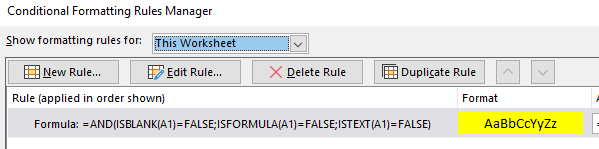In spreadsheets with lots of numbers, sometimes it is useful to see what cells are formulas and what numbers are just hard-defined.

Here is the Conditional-Formatting formula to highlight all the cells with hard-defined numbers (without formulas). Apply this formatting to the whole sheet:

``=AND(ISBLANK(A1)=FALSE;ISFORMULA(A1)=FALSE;ISTEXT(A1)=FALSE)``